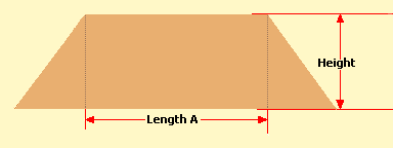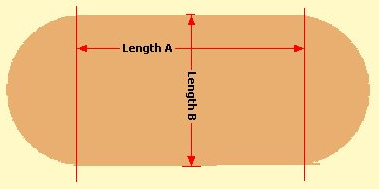Spike's Calculators

# Calculate the Volume of a Pile in Cubic Metres

Estimate the volume of an excavated pile of material in cubic metres.The footprint of this pile looks like this:Length A is not the overall length length of the pile!!

### Calculate the Volume of a Pile

Length A M
Length B M
Height M

#### Results:

 Cubic Metres M³

#### The Calculator

1. Enter the length A in metres
2. Enter the length B in metres
3. Enter the height of the pile in metres

#### The Results

1. The volume of the pile in cubic metres